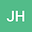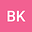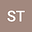Existence of global solutions to a semilinear pseudo-parabolic equation
•••Bhargav KakumaniIn this article, we consider a semilinear pseudo parabolic heat equation with the nonlinearity which is the product of logarithmic and polynomial functions. Here we prove the global existence of solution to the problem for arbitrary dimension $n \geq 1$ and power index $p>1$. Asymptotic behaviour of the solution has been addressed at different energy levels. Moreover, we prove that the global solution indeed decays with an exponential rate. Finally, sufficient conditions are provided under which blow up of solutions take place.